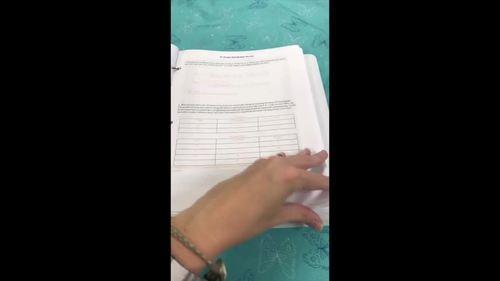# Eureka Grade 6 Study Guides for Modules 1 -6Subject
Resource Type
File Type

Zip

(22 MB|134 pages)
Product Rating
4.0
(5 Ratings)
Standards
• Product Description
• StandardsNEW

This resource provides a study guide for all of the mid-module and end-of-module assessments for Eureka's Grade 6 curriculum. The standards are consistent with those on the assessments.

Use ratio reasoning to convert measurement units; manipulate and transform units appropriately when multiplying or dividing quantities.
Find a percent of a quantity as a rate per 100 (e.g., 30% of a quantity means 30/100 times the quantity); solve problems involving finding the whole, given a part and the percent.
Solve unit rate problems including those involving unit pricing and constant speed. For example, if it took 7 hours to mow 4 lawns, then at that rate, how many lawns could be mowed in 35 hours? At what rate were lawns being mowed?
Make tables of equivalent ratios relating quantities with whole-number measurements, find missing values in the tables, and plot the pairs of values on the coordinate plane. Use tables to compare ratios.
Use ratio and rate reasoning to solve real-world and mathematical problems, e.g., by reasoning about tables of equivalent ratios, tape diagrams, double number line diagrams, or equations.
Total Pages
134 pages
Included
Teaching Duration
N/A
Report this Resource to TpT
Reported resources will be reviewed by our team. Report this resource to let us know if this resource violates TpT’s content guidelines.